# Lab 1: Getting started with the basics¶

As a starting point use `[IasCable](https://github.com/cloud-native-toolkit/iascable)`.

## 1. The `IasCable` framework¶

Let us look at the basic components of the `IasCable` framework.

### Bill of Material and Modules¶

The IasCable uses a `Bill of Material` and `Modules`(from the TechZone Accelerator Toolkit), this is an important point to understand. Bill of Material (BOM) and Modules are the heart of the framework to realize the objective to build an installable component infrastructure based on components from a catalog of available modules.

In a simplified way, we can say a `BOM` is specified by modules it uses, variables you can define and providers you can define. In addition you have the option to use variables and providers definitions related to a `BOM` specification. It is good to know that Modules can have dependencies to other modules, if this is the case the related modules will be included by the framework, as far as I understand.

We can simply say a BOM is specified by modules it uses. In addition you have the option to use variables and providers definitions related to a BOM specification. It is good to know that Modules can have dependencies to other modules, if this is the case, the related modules will be included by the framework, as far as I understand.

Here is a simplified overview diagram of the dependencies: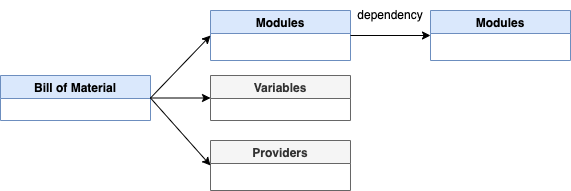Here is a simplified activity diagram that shows the activities carried out by the user and the `IasCable` framework.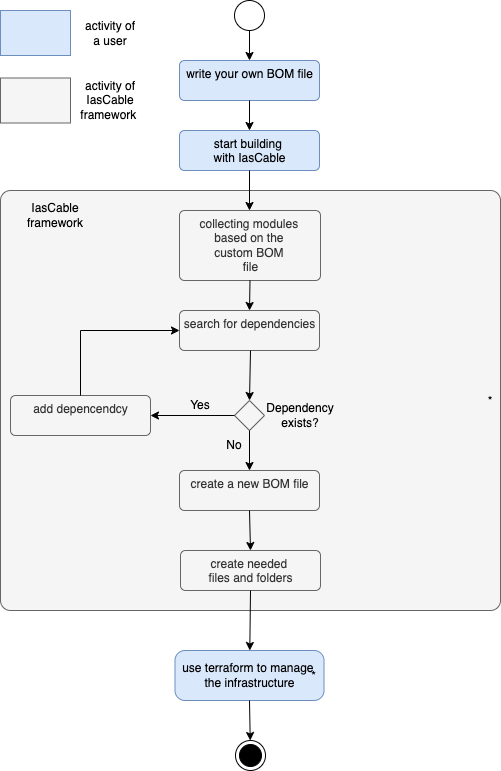### Realize Technology Zone Accelerator Toolkit with Bill of Material and Modules¶

Simplified we can say, we have two basic roles in that context:

1. `Creators` (Architect, Developer or Operator) defining Bill of Materials to create Terraform automation for creating specific infrastructure based on reusing existing Terraform modules.
2. `Consumers` who using the created Terraform automation based on the `BOM` definition.

And we have two major elements to define and create the needed Terraform automation.

1. The `BOM` configures `IasCable` to point to right Terraform modules in a module catalog to create the Terraform automation code.
2. `IasCable` is uses Terraform modules to create a Terraform automation which will be consumed.

The following diagram shows some high level dependencies.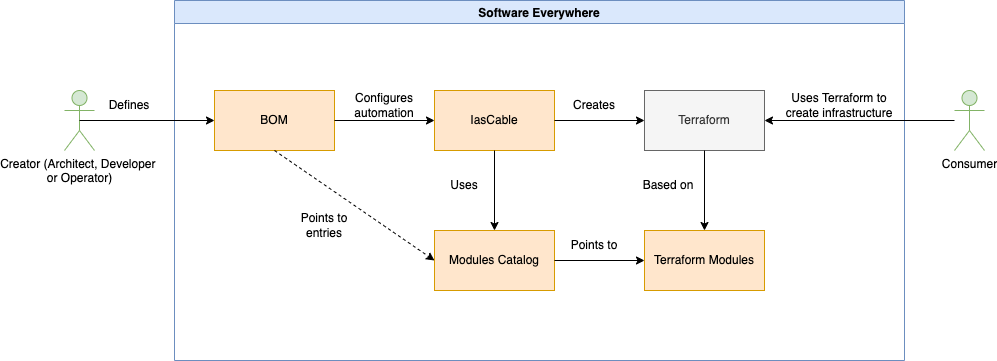## 2. Pre-requisites for the example¶

Following tools need to be installed on your local computer to follow the step by step instructions.

That is the cloud environment we will use.

## 3. `Step-by-step` example setup¶

This is a step by step set up example to create a `Virtual Private Cloud` with three `Subnets` on IBM Cloud.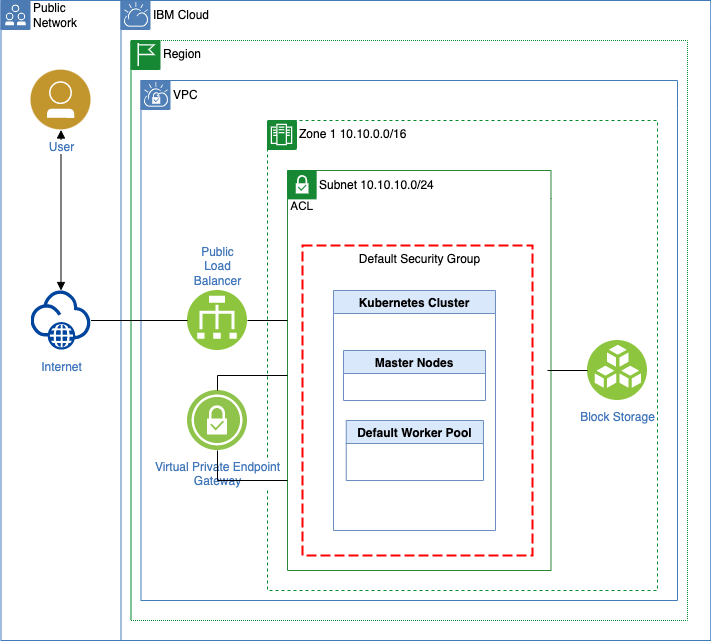### Step 1: Install CLI¶

``````curl -sL https://iascable.cloudnativetoolkit.dev/install.sh | sh
``````

### Step 2: Verify the installation¶

``````iascable build --help
``````
• Example output:
``````Configure (and optionally deploy) the iteration zero assets

Options:
--version                   Show version number   [boolean]
--help                      Show help             [boolean]
-u, --catalogUrl                The url of the module catalog.
Can be https:// or file:/
protocol.
[default: "https://modules.cloudnativetoolkit.dev/index.yaml"]
-i, --input                     The path to the bill of
materials to use as input
[array]
-r, --reference                 The reference BOM to use for
the build               [array]
-o, --outDir                    The base directory where the
command output will be written
[default: "./output"]
--platform                  Filter for the platform
(kubernetes or ocp4)
--provider                  Filter for the provider (ibm or
k8s)
--tileLabel                 The label for the tile.
Required if you want to
--name                      The name used to override the
module name in the bill of
material.               [array]
--tileDescription           The description of the tile.
--flattenOutput, --flatten  Flatten the generated output
into a single directory (i.e.
remove the terraform folder).
[boolean]
--debug                     Flag to turn on more detailed
output message        [boolean]
``````

### Step 3: Create a `Bill of Materials`(BOM) file¶

``````nano firstbom.yaml
``````

Copy following content into the new file:

``````apiVersion: cloudnativetoolkit.dev/v1alpha1
kind: BillOfMaterial
name: ibm-vpc
spec:
modules:
- name: ibm-vpc
- name: ibm-vpc-subnets
``````

### Step 4: Execute following command¶

``````iascable build -i firstbom.yaml
``````

### Step 5: Verify the created content¶

``````├── firstbom.yaml
└── output
├── ibm-vpc
│   ├── apply.sh
│   ├── bom.yaml
│   ├── dependencies.dot
│   ├── destroy.sh
│   └── terraform
│       ├── docs
│       │   ├── ibm-resource-group.md
│       │   ├── ibm-vpc-subnets.md
│       │   └── ibm-vpc.md
│       ├── ibm-vpc.auto.tfvars
│       ├── main.tf
│       ├── providers.tf
│       ├── variables.tf
│       └── version.tf
└── launch.sh
``````
• `output` folder

The folder `output` contains all the content created by the `iascable build` command

• `output/ibm-vpc` folder

The folder `ibm-vpc` is the name we used in our own `BOM` file. Let us call that folder project folder, which was defined in meta data.

``````metadata:
name: ibm-vpc
``````
• `output/ibm-vpc/terraform` folder

This is the table which contains the list of files in the terraform folder.

Filename Content
`output/ibm-vpc/terraform/main.tf` Here you see a number of modules defined including the defined `ibm-vpc` and `ibm-vpc-subnets` from the BOM file.
`output/ibm-vpc/terraform/providers.tf` Simply contains the needed cloud provider information. In that case what we need to specify for `IBM Cloud`.
`output/ibm-vpc/terraform/variables.ft` Contains the specification for the used variable in the `main.tf` or other Terraform files.
`output/ibm-vpc/terraform/version.ft` Contains the specification for the used Terraform provider sources and versions. In that case only IBM is listed.
`output/ibm-vpc/terraform/ibm-vpc.auto.tfvars` That file can be used to configure the variable values. (maybe add to .gitignore) During the execution of `terraform plan` and `terraform apply` you will be ask for input, if you didn't specify that values.
• The `output/launch.sh` file

That script downloads and starts a container on your local machine. The objective is to ensure that the right environment is used for applying the Terraform configuration.

It attaches the local path to the container as a volume.

Note: Need to ensure you have a container engine on your machine. Best Docker! Because by default is uses Docker. Attach doesn't work for `podman` on macOS.

• The `output/ibm-vpc/apply.sh` file

That file converts an existing `variable.yaml` file or variable in the `BOM` file to a `variables.tf` and then executes `terraform init` and `terraform apply`.

• The `output/ibm-vpc/destroy.sh` file

That file simply executes the `terraform init` and `terraform destroy -auto-approve` commands.

• The `output/ibm-vpc/dependencies.dot` file

That file contains the dependencies which can be visualized for example with Graphviz Online.

Example: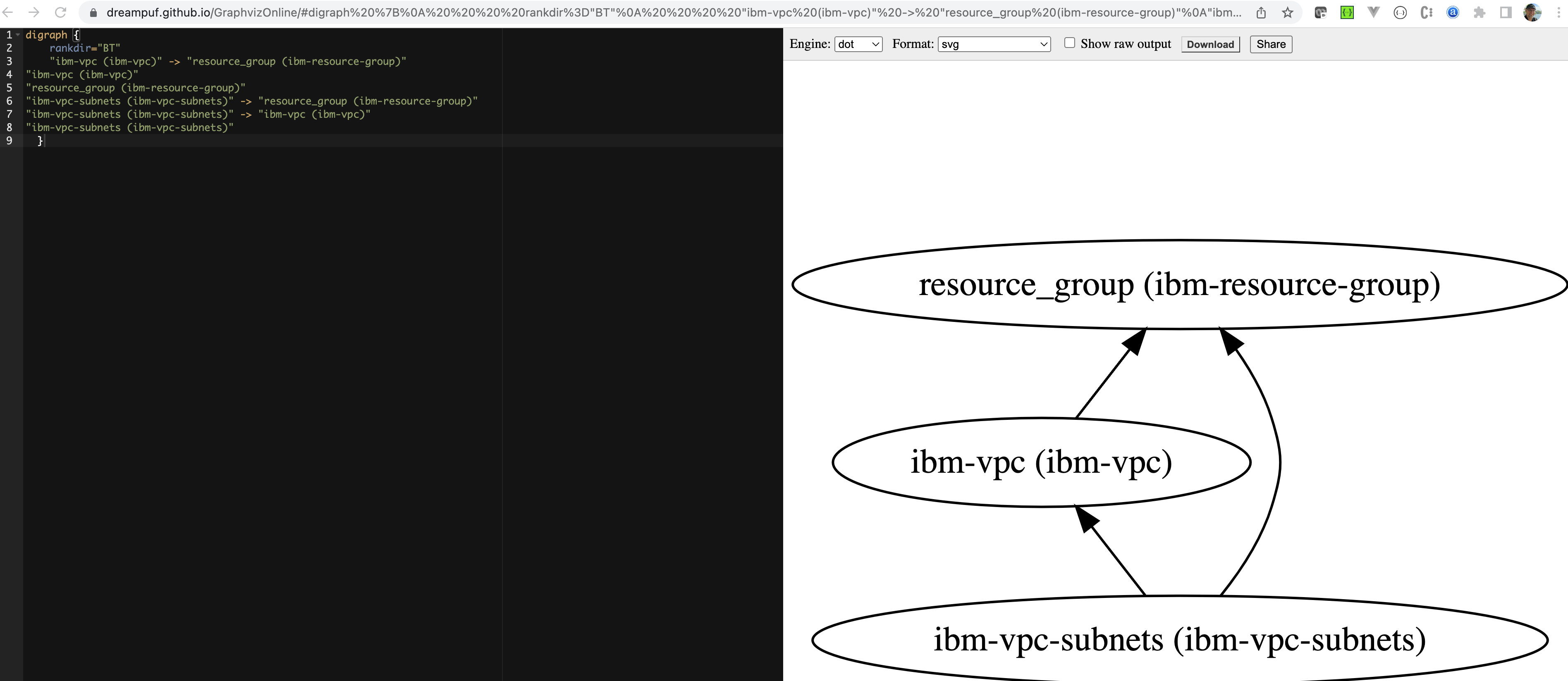• The `output/bom.yaml` file

That file was created by our own `BOM file`. That file now contains all needed variables. These variables are also reflected in the `output/ibm-vpc/terraform/variables.ft` file.

Here is the content of the newly created `bom.yaml` file.

``````apiVersion: cloudnativetoolkit.dev/v1alpha1
kind: BillOfMaterial
name: ibm-vpc
spec:
modules:
- name: ibm-vpc
alias: ibm-vpc
version: v1.16.0
- name: ibm-vpc-subnets
alias: ibm-vpc-subnets
version: v1.13.2
- name: ibm-resource-group
alias: resource_group
version: v3.2.16
variables:
- name: region
type: string
description: The IBM Cloud region where the cluster will be/has been installed.
- name: ibmcloud_api_key
type: string
- name: ibm-vpc-subnets__count
type: number
description: The number of subnets that should be provisioned
defaultValue: 3
- name: resource_group_name
type: string
description: The name of the resource group
``````

### Step 6: Execute the `terraform init` command¶

Navigate to the `output/ibm-vpc/terraform` folder and execute the `terraform init` command.

``````cd output/ibm-vpc/terraform
terraform init
``````

### Step 7: Execute the `terraform plan` command¶

Execute the `terraform plan` command.

``````terraform plan
``````

Here you can see the interaction:

``````var.ibmcloud_api_key
the value of ibmcloud_api_key

Enter a value:
``````

Note: You can create an `IBM Cloud API Key` with following command: `ibmcloud iam api-key-create iascable-example`.

### Step 8: Execute the `terraform apply`¶

Execute the `terraform apply` command.

``````terraform apply -auto-approve
``````
``````var.ibmcloud_api_key
the value of ibmcloud_api_key

Enter a value: xxx
var.region
The IBM Cloud region where the cluster will be/has been installed.

Enter a value: us-south
var.resource_group_name
The name of the resource group

Enter a value: default
``````
• Output overview of the resources which will be created:
``````Terraform used the selected providers to generate the following
execution plan. Resource actions are indicated with the
following symbols:
+ create

Terraform will perform the following actions:

# module.ibm-vpc.data.ibm_is_security_group.base will be read during apply
# (config refers to values not yet known)
<= data "ibm_is_security_group" "base"  {
+ crn                     = (known after apply)
+ id                      = (known after apply)
+ name                    = "default-vpc-base"
+ resource_controller_url = (known after apply)
+ resource_crn            = (known after apply)
+ resource_group_name     = (known after apply)
+ resource_name           = (known after apply)
+ rules                   = (known after apply)
+ tags                    = (known after apply)
+ vpc                     = (known after apply)
}

# module.ibm-vpc.data.ibm_is_vpc.vpc will be read during apply
# (config refers to values not yet known)
<= data "ibm_is_vpc" "vpc"  {
+ classic_access              = (known after apply)
+ crn                         = (known after apply)
+ cse_source_addresses        = (known after apply)
+ default_network_acl         = (known after apply)
+ default_network_acl_crn     = (known after apply)
+ default_network_acl_name    = (known after apply)
+ default_routing_table       = (known after apply)
+ default_routing_table_name  = (known after apply)
+ default_security_group      = (known after apply)
+ default_security_group_crn  = (known after apply)
+ default_security_group_name = (known after apply)
+ id                          = (known after apply)
+ name                        = "default-vpc"
+ resource_controller_url     = (known after apply)
+ resource_crn                = (known after apply)
+ resource_group              = (known after apply)
+ resource_group_name         = (known after apply)
+ resource_name               = (known after apply)
+ resource_status             = (known after apply)
+ security_group              = (known after apply)
+ status                      = (known after apply)
+ subnets                     = (known after apply)
+ tags                        = (known after apply)
}

# module.ibm-vpc.data.ibm_resource_group.resource_group will be read during apply
# (config refers to values not yet known)
<= data "ibm_resource_group" "resource_group"  {
+ account_id          = (known after apply)
+ created_at          = (known after apply)
+ crn                 = (known after apply)
+ id                  = (known after apply)
+ is_default          = (known after apply)
+ name                = "default"
+ payment_methods_url = (known after apply)
+ quota_id            = (known after apply)
+ quota_url           = (known after apply)
+ resource_linkages   = (known after apply)
+ state               = (known after apply)
+ teams_url           = (known after apply)
+ updated_at          = (known after apply)
}

# module.ibm-vpc.ibm_is_network_acl_rule.allow_internal_egress will be created
+ resource "ibm_is_network_acl_rule" "allow_internal_egress" {
+ action      = "allow"
+ before      = (known after apply)
+ destination = "10.0.0.0/8"
+ direction   = "outbound"
+ href        = (known after apply)
+ id          = (known after apply)
+ ip_version  = (known after apply)
+ name        = "allow-internal-egress"
+ network_acl = (known after apply)
+ protocol    = (known after apply)
+ rule_id     = (known after apply)
+ source      = "10.0.0.0/8"
}

# module.ibm-vpc.ibm_is_network_acl_rule.allow_internal_ingress will be created
+ resource "ibm_is_network_acl_rule" "allow_internal_ingress" {
+ action      = "allow"
+ before      = (known after apply)
+ destination = "10.0.0.0/8"
+ direction   = "inbound"
+ href        = (known after apply)
+ id          = (known after apply)
+ ip_version  = (known after apply)
+ name        = "allow-internal-ingress"
+ network_acl = (known after apply)
+ protocol    = (known after apply)
+ rule_id     = (known after apply)
+ source      = "10.0.0.0/8"
}

# module.ibm-vpc.ibm_is_network_acl_rule.deny_external_ingress will be created
+ resource "ibm_is_network_acl_rule" "deny_external_ingress" {
+ action      = "deny"
+ before      = (known after apply)
+ destination = "0.0.0.0/0"
+ direction   = "inbound"
+ href        = (known after apply)
+ id          = (known after apply)
+ ip_version  = (known after apply)
+ name        = "deny-external-ingress"
+ network_acl = (known after apply)
+ protocol    = (known after apply)
+ rule_id     = (known after apply)
+ source      = "0.0.0.0/0"
}

# module.ibm-vpc.ibm_is_network_acl_rule.deny_external_rdp will be created
+ resource "ibm_is_network_acl_rule" "deny_external_rdp" {
+ action      = "deny"
+ before      = (known after apply)
+ destination = "0.0.0.0/0"
+ direction   = "inbound"
+ href        = (known after apply)
+ id          = (known after apply)
+ ip_version  = (known after apply)
+ name        = "deny-external-rdp"
+ network_acl = (known after apply)
+ protocol    = (known after apply)
+ rule_id     = (known after apply)
+ source      = "0.0.0.0/0"

+ tcp {
+ port_max        = 3389
+ port_min        = 3389
+ source_port_max = 3389
+ source_port_min = 3389
}
}

# module.ibm-vpc.ibm_is_network_acl_rule.deny_external_ssh will be created
+ resource "ibm_is_network_acl_rule" "deny_external_ssh" {
+ action      = "deny"
+ before      = (known after apply)
+ destination = "0.0.0.0/0"
+ direction   = "inbound"
+ href        = (known after apply)
+ id          = (known after apply)
+ ip_version  = (known after apply)
+ name        = "deny-external-ssh"
+ network_acl = (known after apply)
+ protocol    = (known after apply)
+ rule_id     = (known after apply)
+ source      = "0.0.0.0/0"

+ tcp {
+ port_max        = 22
+ port_min        = 22
+ source_port_max = 22
+ source_port_min = 22
}
}

# module.ibm-vpc.ibm_is_security_group.base will be created
+ resource "ibm_is_security_group" "base" {
+ crn                     = (known after apply)
+ id                      = (known after apply)
+ name                    = "default-vpc-base"
+ resource_controller_url = (known after apply)
+ resource_crn            = (known after apply)
+ resource_group          = (known after apply)
+ resource_group_name     = (known after apply)
+ resource_name           = (known after apply)
+ rules                   = (known after apply)
+ tags                    = (known after apply)
+ vpc                     = (known after apply)
}

# module.ibm-vpc.ibm_is_security_group_rule.cse_dns_1 will be created
+ resource "ibm_is_security_group_rule" "cse_dns_1" {
+ direction   = "outbound"
+ group       = (known after apply)
+ id          = (known after apply)
+ ip_version  = "ipv4"
+ protocol    = (known after apply)
+ related_crn = (known after apply)
+ remote      = "161.26.0.10"
+ rule_id     = (known after apply)

+ udp {
+ port_max = 53
+ port_min = 53
}
}

# module.ibm-vpc.ibm_is_security_group_rule.cse_dns_1 will be created
+ resource "ibm_is_security_group_rule" "cse_dns_1" {
+ direction   = "outbound"
+ group       = (known after apply)
+ id          = (known after apply)
+ ip_version  = "ipv4"
+ protocol    = (known after apply)
+ related_crn = (known after apply)
+ remote      = "161.26.0.10"
+ rule_id     = (known after apply)

+ udp {
+ port_max = 53
+ port_min = 53
}
}

# module.ibm-vpc.ibm_is_security_group_rule.cse_dns_2 will be created
+ resource "ibm_is_security_group_rule" "cse_dns_2" {
+ direction   = "outbound"
+ group       = (known after apply)
+ id          = (known after apply)
+ ip_version  = "ipv4"
+ protocol    = (known after apply)
+ related_crn = (known after apply)
+ remote      = "161.26.0.11"
+ rule_id     = (known after apply)

+ udp {
+ port_max = 53
+ port_min = 53
}
}

# module.ibm-vpc.ibm_is_security_group_rule.cse_dns_2 will be created
+ resource "ibm_is_security_group_rule" "cse_dns_2" {
+ direction   = "outbound"
+ group       = (known after apply)
+ id          = (known after apply)
+ ip_version  = "ipv4"
+ protocol    = (known after apply)
+ related_crn = (known after apply)
+ remote      = "161.26.0.11"
+ rule_id     = (known after apply)

+ udp {
+ port_max = 53
+ port_min = 53
}
}

# module.ibm-vpc.ibm_is_security_group_rule.default_inbound_http will be created
+ resource "ibm_is_security_group_rule" "default_inbound_http" {
+ direction   = "inbound"
+ group       = (known after apply)
+ id          = (known after apply)
+ ip_version  = "ipv4"
+ protocol    = (known after apply)
+ related_crn = (known after apply)
+ remote      = "0.0.0.0/0"
+ rule_id     = (known after apply)

+ tcp {
+ port_max = 80
+ port_min = 80
}
}

# module.ibm-vpc.ibm_is_security_group_rule.default_inbound_ping will be created
+ resource "ibm_is_security_group_rule" "default_inbound_ping" {
+ direction   = "inbound"
+ group       = (known after apply)
+ id          = (known after apply)
+ ip_version  = "ipv4"
+ protocol    = (known after apply)
+ related_crn = (known after apply)
+ remote      = "0.0.0.0/0"
+ rule_id     = (known after apply)

+ icmp {
+ type = 8
}
}

# module.ibm-vpc.ibm_is_security_group_rule.private_dns_1 will be created
+ resource "ibm_is_security_group_rule" "private_dns_1" {
+ direction   = "outbound"
+ group       = (known after apply)
+ id          = (known after apply)
+ ip_version  = "ipv4"
+ protocol    = (known after apply)
+ related_crn = (known after apply)
+ remote      = "161.26.0.7"
+ rule_id     = (known after apply)

+ udp {
+ port_max = 53
+ port_min = 53
}
}

# module.ibm-vpc.ibm_is_security_group_rule.private_dns_1 will be created
+ resource "ibm_is_security_group_rule" "private_dns_1" {
+ direction   = "outbound"
+ group       = (known after apply)
+ id          = (known after apply)
+ ip_version  = "ipv4"
+ protocol    = (known after apply)
+ related_crn = (known after apply)
+ remote      = "161.26.0.7"
+ rule_id     = (known after apply)

+ udp {
+ port_max = 53
+ port_min = 53
}
}

# module.ibm-vpc.ibm_is_security_group_rule.private_dns_2 will be created
+ resource "ibm_is_security_group_rule" "private_dns_2" {
+ direction   = "outbound"
+ group       = (known after apply)
+ id          = (known after apply)
+ ip_version  = "ipv4"
+ protocol    = (known after apply)
+ related_crn = (known after apply)
+ remote      = "161.26.0.8"
+ rule_id     = (known after apply)

+ udp {
+ port_max = 53
+ port_min = 53
}
}

# module.ibm-vpc.ibm_is_security_group_rule.private_dns_2 will be created
+ resource "ibm_is_security_group_rule" "private_dns_2" {
+ direction   = "outbound"
+ group       = (known after apply)
+ id          = (known after apply)
+ ip_version  = "ipv4"
+ protocol    = (known after apply)
+ related_crn = (known after apply)
+ remote      = "161.26.0.8"
+ rule_id     = (known after apply)

+ udp {
+ port_max = 53
+ port_min = 53
}
}

# module.ibm-vpc.ibm_is_vpc.vpc will be created
+ resource "ibm_is_vpc" "vpc" {
+ classic_access              = false
+ crn                         = (known after apply)
+ cse_source_addresses        = (known after apply)
+ default_network_acl         = (known after apply)
+ default_network_acl_crn     = (known after apply)
+ default_network_acl_name    = "default-vpc-default"
+ default_routing_table       = (known after apply)
+ default_routing_table_name  = "default-vpc-default"
+ default_security_group      = (known after apply)
+ default_security_group_crn  = (known after apply)
+ default_security_group_name = "default-vpc-default"
+ id                          = (known after apply)
+ name                        = "default-vpc"
+ resource_controller_url     = (known after apply)
+ resource_crn                = (known after apply)
+ resource_group              = (known after apply)
+ resource_group_name         = (known after apply)
+ resource_name               = (known after apply)
+ resource_status             = (known after apply)
+ security_group              = (known after apply)
+ status                      = (known after apply)
+ subnets                     = (known after apply)
+ tags                        = (known after apply)
}

# module.ibm-vpc.ibm_resource_tag.nacl-tag will be created
+ resource "ibm_resource_tag" "nacl-tag" {
+ acccount_id = (known after apply)
+ id          = (known after apply)
+ resource_id = (known after apply)
+ tag_type    = (known after apply)
+ tags        = (known after apply)
}

# module.ibm-vpc.ibm_resource_tag.sg-tag will be created
+ resource "ibm_resource_tag" "sg-tag" {
+ acccount_id = (known after apply)
+ id          = (known after apply)
+ resource_id = (known after apply)
+ tag_type    = (known after apply)
+ tags        = (known after apply)
}

# module.ibm-vpc.null_resource.print_names will be created
+ resource "null_resource" "print_names" {
+ id = (known after apply)
}

# module.ibm-vpc-subnets.data.ibm_is_vpc.vpc will be read during apply
# (config refers to values not yet known)
<= data "ibm_is_vpc" "vpc"  {
+ classic_access              = (known after apply)
+ crn                         = (known after apply)
+ cse_source_addresses        = (known after apply)
+ default_network_acl         = (known after apply)
+ default_network_acl_crn     = (known after apply)
+ default_network_acl_name    = (known after apply)
+ default_routing_table       = (known after apply)
+ default_routing_table_name  = (known after apply)
+ default_security_group      = (known after apply)
+ default_security_group_crn  = (known after apply)
+ default_security_group_name = (known after apply)
+ id                          = (known after apply)
+ name                        = "default-vpc"
+ resource_controller_url     = (known after apply)
+ resource_crn                = (known after apply)
+ resource_group              = (known after apply)
+ resource_group_name         = (known after apply)
+ resource_name               = (known after apply)
+ resource_status             = (known after apply)
+ security_group              = (known after apply)
+ status                      = (known after apply)
+ subnets                     = (known after apply)
+ tags                        = (known after apply)
}

# module.ibm-vpc-subnets.data.ibm_resource_group.resource_group will be read during apply
# (config refers to values not yet known)
<= data "ibm_resource_group" "resource_group"  {
+ account_id          = (known after apply)
+ created_at          = (known after apply)
+ crn                 = (known after apply)
+ id                  = (known after apply)
+ is_default          = (known after apply)
+ name                = "default"
+ payment_methods_url = (known after apply)
+ quota_id            = (known after apply)
+ quota_url           = (known after apply)
+ resource_linkages   = (known after apply)
+ state               = (known after apply)
+ teams_url           = (known after apply)
+ updated_at          = (known after apply)
}

# module.ibm-vpc-subnets.ibm_is_network_acl.subnet_acl will be created
+ resource "ibm_is_network_acl" "subnet_acl" {
+ crn                     = (known after apply)
+ id                      = (known after apply)
+ name                    = "default-vpc-subnet-default"
+ resource_controller_url = (known after apply)
+ resource_crn            = (known after apply)
+ resource_group          = (known after apply)
+ resource_group_name     = (known after apply)
+ resource_name           = (known after apply)
+ tags                    = (known after apply)
+ vpc                     = (known after apply)

+ rules {
+ action      = (known after apply)
+ destination = (known after apply)
+ direction   = (known after apply)
+ id          = (known after apply)
+ ip_version  = (known after apply)
+ name        = (known after apply)
+ source      = (known after apply)
+ subnets     = (known after apply)

+ icmp {
+ code = (known after apply)
+ type = (known after apply)
}

+ tcp {
+ port_max        = (known after apply)
+ port_min        = (known after apply)
+ source_port_max = (known after apply)
+ source_port_min = (known after apply)
}

+ udp {
+ port_max        = (known after apply)
+ port_min        = (known after apply)
+ source_port_max = (known after apply)
+ source_port_min = (known after apply)
}
}
}

# module.ibm-vpc-subnets.ibm_is_network_acl_rule.acl_rule will be created
+ resource "ibm_is_network_acl_rule" "acl_rule" {
+ action      = "allow"
+ before      = (known after apply)
+ destination = "10.0.0.0/8"
+ direction   = "inbound"
+ href        = (known after apply)
+ id          = (known after apply)
+ ip_version  = (known after apply)
+ name        = "default-vpc-subnet-default-allow-ingress-internal"
+ network_acl = (known after apply)
+ protocol    = (known after apply)
+ rule_id     = (known after apply)
+ source      = "10.0.0.0/8"
}

# module.ibm-vpc-subnets.ibm_is_network_acl_rule.acl_rule will be created
+ resource "ibm_is_network_acl_rule" "acl_rule" {
+ action      = "allow"
+ before      = (known after apply)
+ destination = "10.0.0.0/8"
+ direction   = "inbound"
+ href        = (known after apply)
+ id          = (known after apply)
+ ip_version  = (known after apply)
+ name        = "default-vpc-subnet-default-allow-roks-ingress"
+ network_acl = (known after apply)
+ protocol    = (known after apply)
+ rule_id     = (known after apply)
+ source      = "166.8.0.0/14"
}

# module.ibm-vpc-subnets.ibm_is_network_acl_rule.acl_rule will be created
+ resource "ibm_is_network_acl_rule" "acl_rule" {
+ action      = "allow"
+ before      = (known after apply)
+ destination = "10.0.0.0/8"
+ direction   = "inbound"
+ href        = (known after apply)
+ id          = (known after apply)
+ ip_version  = (known after apply)
+ name        = "default-vpc-subnet-default-allow-vse-ingress"
+ network_acl = (known after apply)
+ protocol    = (known after apply)
+ rule_id     = (known after apply)
+ source      = "161.26.0.0/16"
}

# module.ibm-vpc-subnets.ibm_is_network_acl_rule.acl_rule will be created
+ resource "ibm_is_network_acl_rule" "acl_rule" {
+ action      = "allow"
+ before      = (known after apply)
+ destination = "10.0.0.0/8"
+ direction   = "outbound"
+ href        = (known after apply)
+ id          = (known after apply)
+ ip_version  = (known after apply)
+ name        = "default-vpc-subnet-default-allow-egress-internal"
+ network_acl = (known after apply)
+ protocol    = (known after apply)
+ rule_id     = (known after apply)
+ source      = "10.0.0.0/8"
}

# module.ibm-vpc-subnets.ibm_is_network_acl_rule.acl_rule will be created
+ resource "ibm_is_network_acl_rule" "acl_rule" {
+ action      = "allow"
+ before      = (known after apply)
+ destination = "166.8.0.0/14"
+ direction   = "outbound"
+ href        = (known after apply)
+ id          = (known after apply)
+ ip_version  = (known after apply)
+ name        = "default-vpc-subnet-default-allow-roks-egress"
+ network_acl = (known after apply)
+ protocol    = (known after apply)
+ rule_id     = (known after apply)
+ source      = "10.0.0.0/8"
}

# module.ibm-vpc-subnets.ibm_is_network_acl_rule.acl_rule will be created
+ resource "ibm_is_network_acl_rule" "acl_rule" {
+ action      = "allow"
+ before      = (known after apply)
+ destination = "161.26.0.0/16"
+ direction   = "outbound"
+ href        = (known after apply)
+ id          = (known after apply)
+ ip_version  = (known after apply)
+ name        = "default-vpc-subnet-default-allow-vse-egress"
+ network_acl = (known after apply)
+ protocol    = (known after apply)
+ rule_id     = (known after apply)
+ source      = "10.0.0.0/8"
}

# module.ibm-vpc-subnets.ibm_is_subnet.vpc_subnets will be created
+ resource "ibm_is_subnet" "vpc_subnets" {
+ access_tags                  = (known after apply)
+ available_ipv4_address_count = (known after apply)
+ crn                          = (known after apply)
+ id                           = (known after apply)
+ ip_version                   = "ipv4"
+ ipv4_cidr_block              = (known after apply)
+ name                         = "default-vpc-subnet-default01"
+ network_acl                  = (known after apply)
+ public_gateway               = (known after apply)
+ resource_controller_url      = (known after apply)
+ resource_crn                 = (known after apply)
+ resource_group               = (known after apply)
+ resource_group_name          = (known after apply)
+ resource_name                = (known after apply)
+ resource_status              = (known after apply)
+ routing_table                = (known after apply)
+ status                       = (known after apply)
+ tags                         = (known after apply)
+ vpc                          = (known after apply)
+ zone                         = "us-south-1"
}

# module.ibm-vpc-subnets.ibm_is_subnet.vpc_subnets will be created
+ resource "ibm_is_subnet" "vpc_subnets" {
+ access_tags                  = (known after apply)
+ available_ipv4_address_count = (known after apply)
+ crn                          = (known after apply)
+ id                           = (known after apply)
+ ip_version                   = "ipv4"
+ ipv4_cidr_block              = (known after apply)
+ name                         = "default-vpc-subnet-default02"
+ network_acl                  = (known after apply)
+ public_gateway               = (known after apply)
+ resource_controller_url      = (known after apply)
+ resource_crn                 = (known after apply)
+ resource_group               = (known after apply)
+ resource_group_name          = (known after apply)
+ resource_name                = (known after apply)
+ resource_status              = (known after apply)
+ routing_table                = (known after apply)
+ status                       = (known after apply)
+ tags                         = (known after apply)
+ vpc                          = (known after apply)
+ zone                         = "us-south-2"
}

# module.ibm-vpc-subnets.ibm_is_subnet.vpc_subnets will be created
+ resource "ibm_is_subnet" "vpc_subnets" {
+ access_tags                  = (known after apply)
+ available_ipv4_address_count = (known after apply)
+ crn                          = (known after apply)
+ id                           = (known after apply)
+ ip_version                   = "ipv4"
+ ipv4_cidr_block              = (known after apply)
+ name                         = "default-vpc-subnet-default03"
+ network_acl                  = (known after apply)
+ public_gateway               = (known after apply)
+ resource_controller_url      = (known after apply)
+ resource_crn                 = (known after apply)
+ resource_group               = (known after apply)
+ resource_group_name          = (known after apply)
+ resource_name                = (known after apply)
+ resource_status              = (known after apply)
+ routing_table                = (known after apply)
+ status                       = (known after apply)
+ tags                         = (known after apply)
+ vpc                          = (known after apply)
+ zone                         = "us-south-3"
}

# module.ibm-vpc-subnets.null_resource.print_names will be created
+ resource "null_resource" "print_names" {
+ id = (known after apply)
}

# module.resource_group.data.ibm_resource_group.resource_group will be read during apply
# (config refers to values not yet known)
<= data "ibm_resource_group" "resource_group"  {
+ account_id          = (known after apply)
+ created_at          = (known after apply)
+ crn                 = (known after apply)
+ id                  = (known after apply)
+ is_default          = (known after apply)
+ name                = "default"
+ payment_methods_url = (known after apply)
+ quota_id            = (known after apply)
+ quota_url           = (known after apply)
+ resource_linkages   = (known after apply)
+ state               = (known after apply)
+ teams_url           = (known after apply)
+ updated_at          = (known after apply)
}

# module.resource_group.data.ibm_resource_tag.resource_group_tags will be read during apply
# (config refers to values not yet known)
<= data "ibm_resource_tag" "resource_group_tags"  {
+ id          = (known after apply)
+ resource_id = (known after apply)
+ tags        = (known after apply)
}

# module.resource_group.null_resource.resource_group will be created
+ resource "null_resource" "resource_group" {
+ id       = (known after apply)
+ triggers = (known after apply)
}

# module.resource_group.null_resource.wait_for_sync will be created
+ resource "null_resource" "wait_for_sync" {
+ id = (known after apply)
}

# module.resource_group.random_uuid.tag will be created
+ resource "random_uuid" "tag" {
+ id     = (known after apply)
+ result = (known after apply)
}

# module.resource_group.module.clis.data.external.setup-binaries will be read during apply
# (config refers to values not yet known)
<= data "external" "setup-binaries"  {
+ id      = (known after apply)
+ program = [
+ "bash",
+ ".terraform/modules/resource_group.clis/scripts/setup-binaries.sh",
]
+ query   = {
+ "clis"    = "yq,jq,igc"
+ "uuid"    = (known after apply)
}
+ result  = (known after apply)
}

# module.resource_group.module.clis.null_resource.print will be created
+ resource "null_resource" "print" {
+ id = (known after apply)
}

# module.resource_group.module.clis.random_string.uuid will be created
+ resource "random_string" "uuid" {
+ id          = (known after apply)
+ length      = 16
+ lower       = true
+ min_lower   = 0
+ min_numeric = 0
+ min_special = 0
+ min_upper   = 0
+ number      = false
+ numeric     = (known after apply)
+ result      = (known after apply)
+ special     = false
+ upper       = false
}

Plan: 36 to add, 0 to change, 0 to destroy.
╷
│ Warning: Experimental feature "module_variable_optional_attrs" is active
│
│   on .terraform/modules/ibm-vpc-subnets/version.tf line 10, in terraform:
│   10:   experiments = [module_variable_optional_attrs]
│
│ Experimental features are subject to breaking changes in
│ future minor or patch releases, based on feedback.
│
│ If you have feedback on the design of this feature, please
│ open a GitHub issue to discuss it.
╵
╷
│ Warning: Argument is deprecated
│
│   with module.resource_group.module.clis.random_string.uuid,
│   on .terraform/modules/resource_group.clis/main.tf line 15, in resource "random_string" "uuid":
│   15:   number  = false
│
│
│ (and one more similar warning elsewhere)
Do you want to perform these actions?
Terraform will perform the actions described above.
Only 'yes' will be accepted to approve.

Enter a value: yes
``````
• Final result of the creation
``````Apply complete! Resources: 36 added, 0 changed, 0 destroyed.
``````

### Step 9: Execute the `terraform destroy` command¶

Note: Ensure you didn't delete the `terraform.tfstate` and the `.terraform.lock.hcl` files before.

To destroy the provisioned resources, run the following:

``````terraform destroy -auto-approve
``````

You need to ensure to provide the IBM Cloud API Key, the region and the resource group name.

``````var.ibmcloud_api_key
the value of ibmcloud_api_key

Enter a value: xxxx

var.region
The IBM Cloud region where the cluster will be/has been installed.

Enter a value: us-south

var.resource_group_name
The name of the resource group

Enter a value: default
``````
• Output overview:
``````╷
│ Warning: Experimental feature "module_variable_optional_attrs" is active
│
│   on .terraform/modules/ibm-vpc-subnets/version.tf line 10, in terraform:
│   10:   experiments = [module_variable_optional_attrs]
│
│ Experimental features are subject to breaking changes in
│ future minor or patch releases, based on feedback.
│
│ If you have feedback on the design of this feature, please
│ open a GitHub issue to discuss it.
╵
╷
│ Warning: Argument is deprecated
│
│   with module.resource_group.module.clis.random_string.uuid,
│   on .terraform/modules/resource_group.clis/main.tf line 15, in resource "random_string" "uuid":
│   15:   number  = false
│
The `IasCable` and the `Modules` (from the TechZone Accelerator Toolkit) are all from the Cloud Native Toolkit providing a good concept for a framework. This helps to provide reusable components to install and configure cloud infrastructure. What you have learned so far is just helping you to get started, there is more to learn.